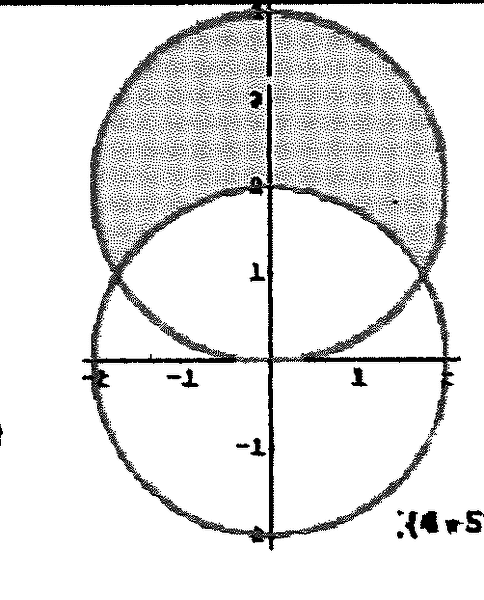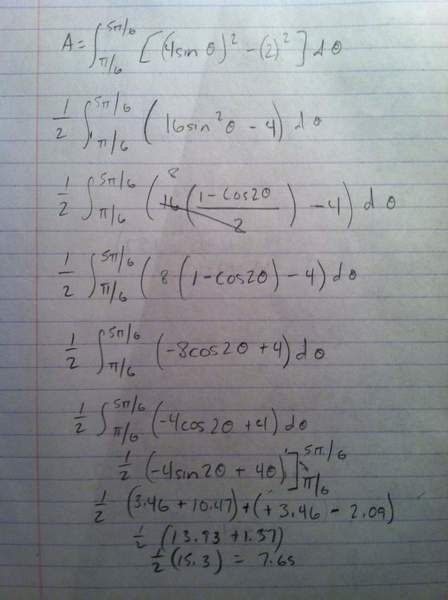# Polar coordinates

queenstudy
i just need to know something about the integral with polar coordinates , to know the interval of the teta angle of any domain the proffesor said that we put the pen on the x-axis and move it , i for one moment was not focusing and the doctor had to go to another class can any one explain ??? thank you

## Answers and Replies

Homework Helper
Well, I have no clue what is meant here, or even what question you are asking. Perhaps he/she was saying that the angle, for a point (x,y) in Cartesian coordinates, is the angle the line through (0, 0) and (x, y) makes with the x-axis. That is, you measure the angle from the x-axis to that line.

queenstudy
i mean if you have a graph and you want to change your coordinates from cartesian to polar so that the double intergral is easier
okay here is an example a semi circle of center O(2,0) if i want to find teta in polar coordinates it will be 90 degree why

bbjb
Any help is much appreciated

https://www.physicsforums.com/attachments/41642

(5pi/6)
A= 1/2 ∫ [(4 sin⁡θ )^2-(2)^2 ]dθ
(pi/6)

4 sin⁡θ=2 θ=π/6 ,5π/6

Homework Helper
What, exactly, are you asking? How they got those limits of integration?

You have two circles, one with center at (0, 0) and radius 2 and so equation $x^2+ y^2= 4$, the other with center at (0, 2) and radius 2 and so equation $x^2+ (y- 2)^2= x^2+ y^2- 4y+ 4= 4$. Since those are both equal to 4, they are equal to each other: $x^2+ y^2= x^2+ y^2- 4y+ 4$ which reduces to 4y= 4 and y= 1. Then we have $x^2+ y^2= x^2+ 1= 4$ so $x^2= 3$ and $x= \pm\sqrt{3}$.
The two points of intersection are $(\sqrt{3}, 1)$, $(-\sqrt{3}, 1)$.

In terms of polar coordinates, $\theta= tan^{-1}(y/x)$ which gives $\theta= tan^{-1}(1/\sqrt{3})= \pi/6$ and $\theta= tan^{-1}(-1/\sqrt{3})= 5\pi/6$.

bbjb
Thanks for replying back soo fast I appreciate it i was trying to find the shade parts are in attachment I also think I have it figured out but wouldnt mind a second pair of eyes to look over it. See attachmentsThanks again

queenstudy
ok nice exercise now i got it

How did the "$-8 cos(2\theta)+ 4$" on the fifth line become "$-4 cos(2\theta)+ 4$" on the sixth line?
On the very next line, after integrating you have $-4 sin(2\theta)+ 4\theta$ which is correct because the integral of $cos(2\theta)$ is $(sin(2\theta))(1/2)$ but there is no reason to divide by 2 before the integration.